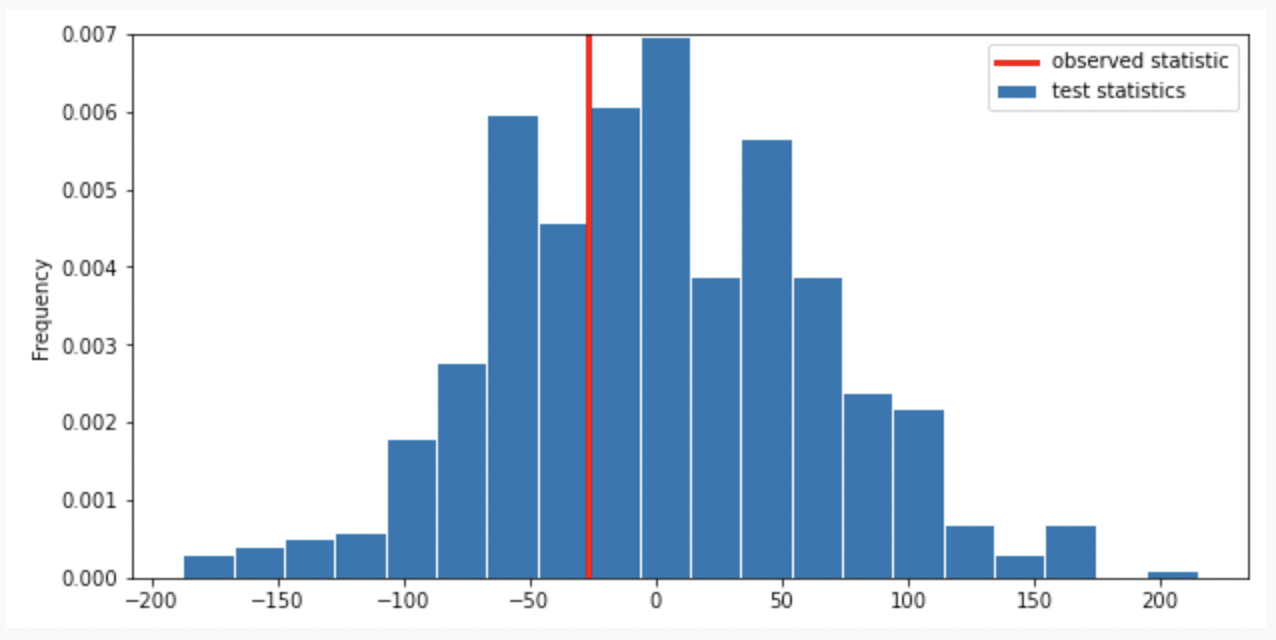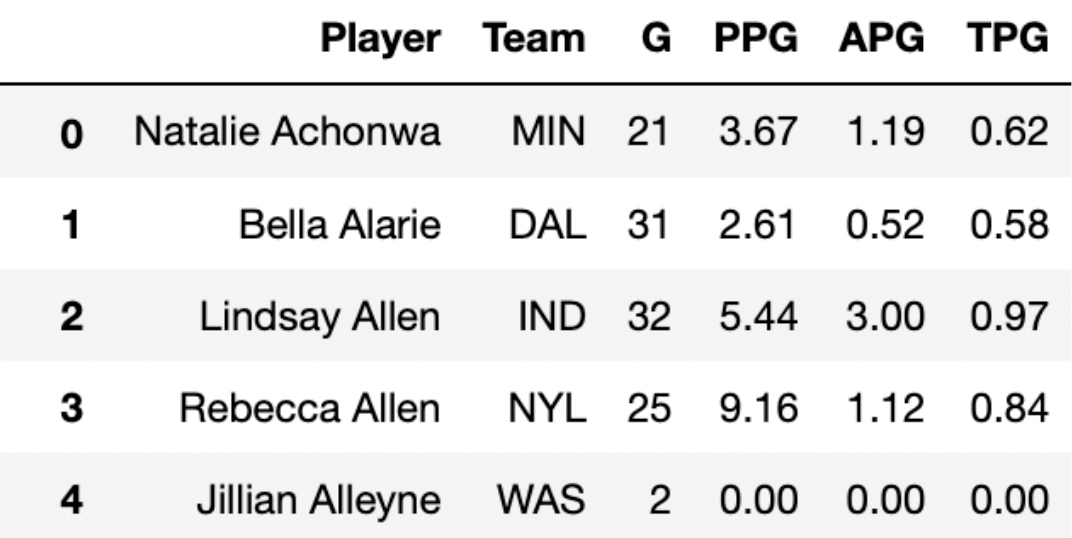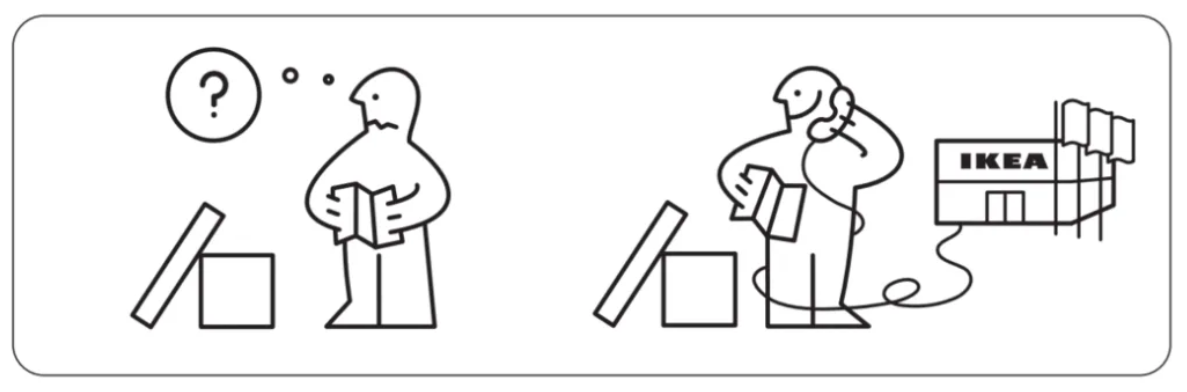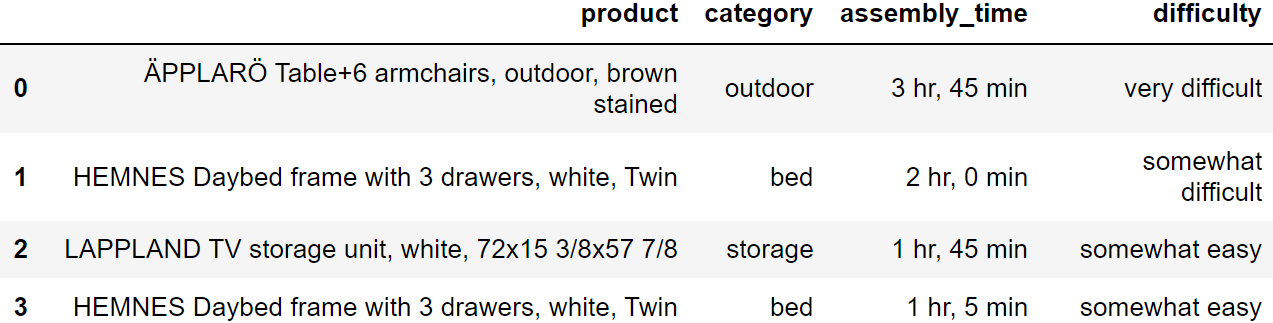# Discussion 8: Permutation Testing and Bootstrapping

The problems in this worksheet are taken from past exams. Work on them on paper, since the exams you take in this course will also be on paper.

We encourage you to complete this worksheet in a live discussion section. Solutions will be made available after all discussion sections have concluded. You don’t need to submit your answers anywhere.

Note: We do not plan to cover all problems here in the live discussion section; the problems we don’t cover can be used for extra practice.

## Problem 1

Researchers from the San Diego Zoo, located within Balboa Park, collected physical measurements of three species of penguins (Adelie, Chinstrap, or Gentoo) in a region of Antarctica. One piece of information they tracked for each of 330 penguins was its mass in grams. The average penguin mass is 4200 grams, and the standard deviation is 840 grams.

We’re interested in investigating the differences between the masses of Adelie penguins and Chinstrap penguins. Specifically, our null hypothesis is that their masses are drawn from the same population distribution, and any observed differences are due to chance only.

Below, we have a snippet of working code for this hypothesis test, for a specific test statistic. Assume that adelie_chinstrap is a DataFrame of only Adelie and Chinstrap penguins, with just two columns – 'species' and 'mass'.

stats = np.array([])
num_reps = 500
for i in np.arange(num_reps):
# --- line (a) starts ---
# --- line (a) ends ---

# --- line (b) starts ---
# --- line (b) ends ---

grouped = with_shuffled.groupby('species').mean()

# --- line (c) starts ---
stat = grouped.get('mass').iloc - grouped.get('mass').iloc
# --- line (c) ends ---

stats = np.append(stats, stat)

### Problem 1.1

Which of the following statements best describe the procedure above?

• This is a standard hypothesis test, and our test statistic is the total variation distance between the distribution of Adelie masses and Chinstrap masses

• This is a standard hypothesis test, and our test statistic is the difference between the expected proportion of Adelie penguins and the proportion of Adelie penguins in our resample

• This is a permutation test, and our test statistic is the total variation distance between the distribution of Adelie masses and Chinstrap masses

• This is a permutation test, and our test statistic is the difference in the mean Adelie mass and mean Chinstrap mass

### Problem 1.2

Currently, line (c) (marked with a comment) uses .iloc. Which of the following options compute the exact same statistic as line (c) currently does?

Option 1:

stat = grouped.get('mass').loc['Adelie'] - grouped.get('mass').loc['Chinstrap']

Option 2:

stat = grouped.get('mass').loc['Chinstrap'] - grouped.get('mass').loc['Adelie']
• Option 1 only

• Option 2 only

• Both options

• Neither option

### Problem 1.3

Is it possible to re-write line (c) in a way that uses .iloc twice, without any other uses of .loc or .iloc?

• Yes, it’s possible

• No, it’s not possible

### Problem 1.4

For your convenience, we copy the code for the hypothesis test below.

stats = np.array([])
num_reps = 500
for i in np.arange(num_reps):
# --- line (a) starts ---
# --- line (a) ends ---

# --- line (b) starts ---
# --- line (b) ends ---

grouped = with_shuffled.groupby('species').mean()

# --- line (c) starts ---
stat = grouped.get('mass').iloc - grouped.get('mass').iloc
# --- line (c) ends ---

stats = np.append(stats, stat)

What would happen if we removed line (a), and replaced line (b) with

with_shuffled = adelie_chinstrap.sample(adelie_chinstrap.shape, replace=False)

• This would still run a valid hypothesis test

• This would not run a valid hypothesis test, as all values in the stats array would be exactly the same

• This would not run a valid hypothesis test, even though there would be several different values in the stats array

• This would not run a valid hypothesis test, as it would incorporate information about Gentoo penguins

### Problem 1.5

For your convenience, we copy the code for the hypothesis test below.

stats = np.array([])
num_reps = 500
for i in np.arange(num_reps):
# --- line (a) starts ---
# --- line (a) ends ---

# --- line (b) starts ---
# --- line (b) ends ---

grouped = with_shuffled.groupby('species').mean()

# --- line (c) starts ---
stat = grouped.get('mass').iloc - grouped.get('mass').iloc
# --- line (c) ends ---

stats = np.append(stats, stat)

What would happen if we removed line (a), and replaced line (b) with

with_shuffled = adelie_chinstrap.sample(adelie_chinstrap.shape, replace=True)

• This would still run a valid hypothesis test

• This would not run a valid hypothesis test, as all values in the stats array would be exactly the same

• This would not run a valid hypothesis test, even though there would be several different values in the stats array

• This would not run a valid hypothesis test, as it would incorporate information about Gentoo penguins

### Problem 1.6

For your convenience, we copy the code for the hypothesis test below.

stats = np.array([])
num_reps = 500
for i in np.arange(num_reps):
# --- line (a) starts ---
# --- line (a) ends ---

# --- line (b) starts ---
# --- line (b) ends ---

grouped = with_shuffled.groupby('species').mean()

# --- line (c) starts ---
stat = grouped.get('mass').iloc - grouped.get('mass').iloc
# --- line (c) ends ---

stats = np.append(stats, stat)

What would happen if we replaced line (a) with

with_shuffled = adelie_chinstrap.assign(
)

and replaced line (b) with

with_shuffled = with_shuffled.assign(
)

• This would still run a valid hypothesis test

• This would not run a valid hypothesis test, as all values in the stats array would be exactly the same

• This would not run a valid hypothesis test, even though there would be several different values in the stats array

• This would not run a valid hypothesis test, as it would incorporate information about Gentoo penguins

### Problem 1.7

Suppose we run the code for the hypothesis test and see the following empirical distribution for the test statistic. In red is the observed statistic.Suppose our alternative hypothesis is that Chinstrap penguins weigh more on average than Adelie penguins. Which of the following is closest to the p-value for our hypothesis test?

• 0

• \frac{1}{4}

• \frac{1}{3}

• \frac{2}{3}

• \frac{3}{4}

• 1

## Problem 2

For this question we will use data from the 2021 Women’s National Basketball Association (WNBA) season for the next several problems. In basketball, players score points by shooting the ball into a hoop. The team that scores the most points wins the game.

We have access to the season DataFrame, which contains statistics on all players in the WNBA in the 2021 season. The first few rows of season are shown below.Each row in season corresponds to a single player. For each player, we have: - 'Player' (str), their name - 'Team' (str), the three-letter code of the team they play on - 'G' (int), the number of games they played in the 2021 season - 'PPG' (float), the number of points they scored per game played - 'APG' (float), the number of assists (passes) they made per game played - 'TPG' (float), the number of turnovers they made per game played

Note that all of the numerical columns in season must contain values that are greater than or equal to 0.

Suppose we only have access to the DataFrame small_season, which is a random sample of size 36 from season. We’re interested in learning about the true mean points per game of all players in season given just the information in small_season.

To start, we want to bootstrap small_season 10,000 times and compute the mean of the resample each time. We want to store these 10,000 bootstrapped means in the array boot_means.

Here is a broken implementation of this procedure.

boot_means = np.array([])
for i in np.arange(10000):
resample = small_season.sample(season.shape, replace=False)  # Line 1
resample_mean = small_season.get('PPG').mean()                  # Line 2
np.append(boot_means, new_mean)                                 # Line 3

For each of the 3 lines of code above (marked by comments), specify what is incorrect about the line by selecting one or more of the corresponding options below. Or, select “Line _ is correct as-is” if you believe there’s nothing that needs to be changed about the line in order for the above code to run properly.

### Problem 2.1

What is incorrect about Line 1? Select all that apply.

• Currently the procedure samples from small_season, when it should be sampling from season

• The sample size is season.shape, when it should be small_season.shape

• Sampling is currently being done without replacement, when it should be done with replacement

• Line 1 is correct as-is

### Problem 2.2

What is incorrect about Line 2? Select all that apply.

• Currently it is taking the mean of the 'PPG' column in small_season, when it should be taking the mean of the 'PPG' column in season

• Currently it is taking the mean of the 'PPG' column in small_season, when it should be taking the mean of the 'PPG' column in resample

• .mean() is not a valid Series method, and should be replaced with a call to the function np.mean

• Line 2 is correct as-is

### Problem 2.3

What is incorrect about Line 3? Select all that apply.

• The result of calling np.append is not being reassigned to boot_means, so boot_means will be an empty array after running this procedure

• The indentation level of the line is incorrect – np.append should be outside of the for-loop (and aligned with for i)

• new_mean is not a defined variable name, and should be replaced with resample_mean

• Line 3 is correct as-is

## Problem 3

IKEA is a Swedish furniture company that designs and sells ready-to-assemble furniture and other home furnishings.An IKEA fan created an app where people can log the amount of time it took them to assemble their IKEA furniture. The DataFrame app_data has a row for each product build that was logged on the app. The columns are:

• 'product' (str): the name of the product, which includes the product line as the first word, followed by a description of the product
• 'category' (str): a categorical description of the type of product
• 'assembly_time' (str): the amount of time to assemble the product, formatted as 'x hr, y min' where x and y represent integers, possibly zero

The first few rows of app_data are shown below, though app_data has many more rows than pictured (5000 rows total).Assume that we have already run import babypandas as bpd and import numpy as np.

We want to use app_data to estimate the average amount of time it takes to build an IKEA bed (any product in the ‘bed’ category). Which of the following strategies would be an appropriate way to estimate this quantity? Select all that apply.

• Query to keep only the beds. Then resample with replacement many times. For each resample, take the mean of the 'minutes' column. Compute a 95% confidence interval based on those means.

• Query to keep only the beds. Group by 'product' using the mean aggregation function. Then resample with replacement many times. For each resample, take the mean of the 'minutes' column. Compute a 95% confidence interval based on those means.

• Resample with replacement many times. For each resample, first query to keep only the beds and then take the mean of the 'minutes' column. Compute a 95% confidence interval based on those means.

• Resample with replacement many times. For each resample, first query to keep only the beds. Then group by 'product' using the mean aggregation function, and finally take the mean of the 'minutes' column. Compute a 95% confidence interval based on those means.

## Problem 4

True or False: Suppose that from a sample, you compute a 95% bootstrapped confidence interval for a population parameter to be the interval [L, R]. Then the average of L and R is the mean of the original sample.

## Problem 5

Suppose Tiffany has a random sample of dogs. Select the most appropriate technique to answer each of the following questions using Tiffany’s dog sample.

### Problem 5.1

Do small dogs typically live longer than medium and large dogs?

• Standard hypothesis test

• Permutation test

• Bootstrapping

### Problem 5.2

Does Tiffany’s sample have an even distribution of dog kinds?

• Standard hypothesis test

• Permutation test

• Bootstrapping

### Problem 5.3

What’s the median weight for herding dogs?

• Standard hypothesis test

• Permutation test

• Bootstrapping

### Problem 5.4

Do dogs live longer than 12 years on average?

• Standard hypothesis test

• Permutation test

• Bootstrapping